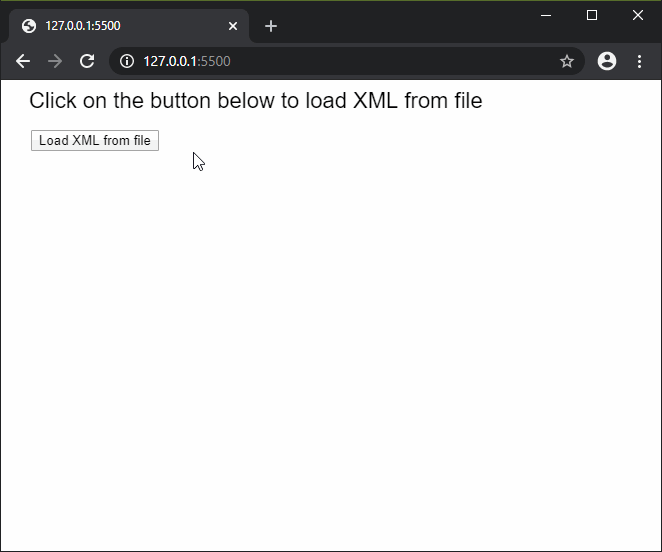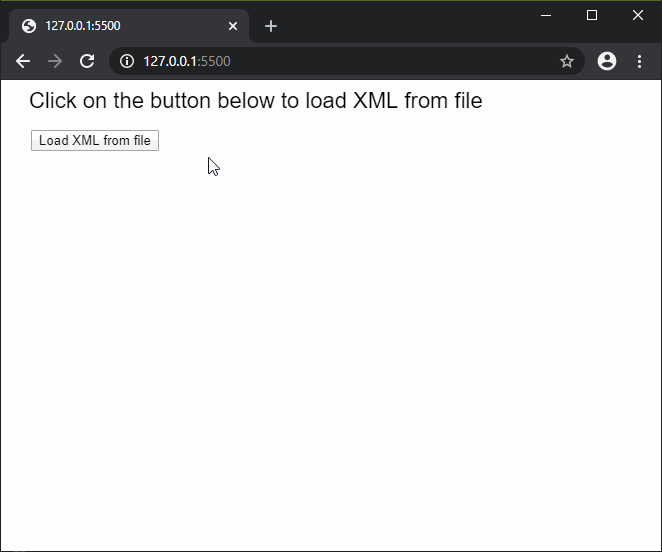The loadXML() function is used to read the contents of a file or URL and return it as a XML object. The file must be present in the sketch directory to be accessed. This method can be used to support file sizes up to 64MB.

This function is asynchronous, therefore it is recommended to be called in the preload() function to ensure that the function is executed before the other functions.

Syntax:

`loadXML(filename, callback, errorCallback )`

Parameters: This function accepts three parameters as mentioned above and described below:

• filename: This is a string which denotes the file path or URL from where the XML data has to be loaded.
• callback: This is a function which is called when this function executes successfully. The first argument for this function is the XML data loaded from the file. It is an optional parameter.
• errorCallback: This is a function which is called if there is any error in executing the function. The first argument for this function is the error response. It is an optional parameter.

The examples below illustrate the loadXML() function in p5.js:

Example 1:

 `/* == Contents of books.xml == ` ` `  ` ` `    ``The Adventures of Sherlock Holmes: Part One ` `    ``Arthur Conan Doyle ` `    ``323 ` `    ``Detective fiction ` ` ` ` `  `*/` ` `  `let loadedXML = ``null``; ` ` `  `function` `setup() { ` `  ``createCanvas(500, 200); ` `  ``textSize(22); ` ` `  `  ``text(``"Click on the button below to "` `       ``+ ``"load XML from file"``, 20, 20); ` ` `  `  ``// Create a button for loading the XML ` `  ``loadBtn = createButton(``"Load XML from file"``); ` `  ``loadBtn.position(30, 50) ` `  ``loadBtn.mousePressed(loadXMLFile); ` `} ` ` `  `function` `loadXMLFile() { ` ` `  `  ``// Load the XML from file ` `  ``loadedXML = loadXML(``'books.xml'``, onFileload); ` `} ` ` `  `function` `onFileload() { ` `  ``text(``"XML loaded successfully..."``, 30, 100); ` `  ``let book = loadedXML.getChildren(); ` `   `  `  ``// Get the content of the tags ` `  ``let name = book.getContent(); ` `  ``let author = book.getContent(); ` `  ``let price = book.getContent(); ` `  ``let genre = book.getContent(); ` ` `  `  ``text(``"Name: "` `+ name, 30, 140); ` `  ``text(``"Author: "` `+ author, 30, 160); ` `  ``text(``"Price: "` `+ price, 30, 180); ` `  ``text(``"Genre: "` `+ genre, 30, 200); ` `} `

Output:Example 2:

 `/* == Contents of movies.xml == ` ` `  ` ` `    `` ` `        ``The Godfather ` `    `` ` `    `` ` `        ``The Wizard of Oz ` `    `` ` `    `` ` `        ``Citizen Kane ` `    `` ` ` ` ` `  `*/` ` `  `let loadedXML = ``null``; ` ` `  `function` `setup() { ` `  ``createCanvas(500, 450); ` `  ``textSize(22); ` ` `  `  ``text(``"Click on the button below to "` `        ``+ ``"load XML from file"``, 20, 20); ` ` `  `  ``// Create a button for loading the XML ` `  ``loadBtn = createButton(``"Load XML from file"``); ` `  ``loadBtn.position(30, 50) ` `  ``loadBtn.mousePressed(loadXMLFile); ` `} ` ` `  `function` `loadXMLFile() { ` ` `  `  ``// Load the XML from file ` `  ``loadedXML = loadXML(``'movies.xml'``, onFileload); ` `} ` ` `  `function` `onFileload() { ` ` `  `  ``// Get the children with the "movie" tag ` `  ``let children = loadedXML.getChildren(``'movie'``); ` ` `  `  ``for` `(let i = 0; i < children.length; i++) { ` ` `  `    ``// Get the content of the tag ` `    ``let name = children[i].getContent(); ` ` `  `    ``// Get a numerical attribute ` `    ``let year = children[i].getNum(``'year'``); ` ` `  `    ``// Get a string attribute ` `    ``let director = children[i].getString(``'director'``); ` ` `  `    ``text(``"Name: "` `+ name, 30, 100 + i * 80); ` `    ``text(``"Year: "` `+ year, 30, 120 + i * 80); ` `    ``text(``"Director: "` `+ director, 30, 140 + i * 80); ` `  ``} ` `} `

Output:Online editor: https://editor.p5js.org/My Personal Notes arrow_drop_upCheck out this Author's contributed articles.

If you like GeeksforGeeks and would like to contribute, you can also write an article using contribute.geeksforgeeks.org or mail your article to contribute@geeksforgeeks.org. See your article appearing on the GeeksforGeeks main page and help other Geeks.

Please Improve this article if you find anything incorrect by clicking on the "Improve Article" button below.

Article Tags :

Be the First to upvote.

Please write to us at contribute@geeksforgeeks.org to report any issue with the above content.